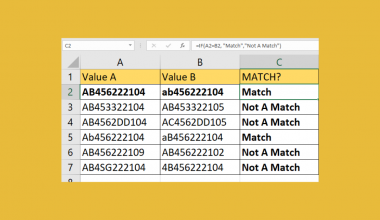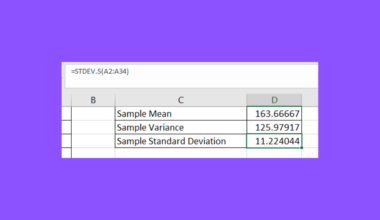# How to Do 3D Interpolation in Excel

This guide will explain how to do 3D interpolation in Excel.

Linear interpolation refers to a method used to estimate values between two known terms. This technique is often used when data points are missing between known values.

While the simplest form of linear interpolation looks for values between two points in a 2D grid, we can extend the technique to handle 3D points as well.

We can use custom Excel formulas to calculate an interpolated point between two given 3D points.

In this guide, we will provide a step-by-step tutorial on how to perform 3D interpolation in Excel.

## A Real Example of 3D Interpolation in Excel

Given two points A(x1, y1, z1) and B(x2, y2, z2), we are interested in finding the interpolated point P(x, y, z) that exists along the line segment connecting A and B.

We’ll use the variable t to refer to the fractional distance along that line segment. For example, if we want to find the interpolated point halfway through the line connecting A and B, we’ll just set t=0.5.

We can find the values for x, y, and z using the following formulas:

`x = x1+t(x2 -x1)`
`y = y1+t(y2 -y1)`
`z = z1+t(z2 -z1)`

The formulas above assume that t=0 is equivalent to point A and t=1 is equivalent to point B.

### Finding an Interpolated Point Between Two 3D Points

Let’s take a look at a sample use case where we’ll use linear interpolation given 3D coordinates.

In the table above, we have entered the x,y, and z values of points A and B. We want to determine the midpoint between these two values, which is the interpolated point exactly halfway through the line connecting these two points.

To find the x value of the midpoint, we’ll use the following Excel formula:

`=B2+B5*(B3-B2)`

The formula above uses the linear interpolation formula described earlier. We’ve added the value 0.5 in cell B5 to indicate that we want to find the midpoint.

To find the y and z values, we’ll just need to modify our formula to use the y-coordinates and z-coordinates of our points.

For our midpoint’s y-value, we’ll use the formula =C2+B5*(C3-C2), and we’ll use the formula =D2+B5*(D3-D2) for our z-value.

We can modify the value of t to determine the interpolated point at some fractional distance between points A and B.

In the example above, we’ve set the value of t to 0.25 to find the coordinates of the interpolated point located a quarter of the total distance from point A.

Click on the link below to create your own copy of our examples.

Head to the next section to read our step-by-step tutorial on how to start performing linear interpolation on 3D points.

## How to Do 3D Interpolation in Excel

1. Before performing linear interpolation, we need to identify the coordinates of our starting point A and ending point. We will also need to determine the fractional distance that will determine where between these two points we will interpolate.In our example, we’ll use the points A(5.63, 9.21, 3.12) and B(11.33, 8.23, 4.5) and the fractional distance of 0.25.
2. Next, we’ll compute the x-coordinate of our interpolated point. We’ll use the formula =B2+B5*(B3-B2).3. Hit the Enter key to evaluate the formula.In the table above, we’ve determined that our interpolated point has an x-coordinate of 7.055.
4. Next, we’ll need to compute the y-coordinate of our interpolated point. We’ll use the formula =C2+B5*(C3-C2).Our interpolated point’s y-coordinate is 8.965.
5. Next, we’ll need to compute the z-coordinate of our interpolated point. We’ll use the formula =D2+B5*(D3-D2).After finding all three coordinates, we’ve now determined our interpolated point P(7.055 ,8.965, 3.465)

These are all the steps you need to start performing 3D interpolation in Microsoft Excel.

## FAQs

1. Why might I need to use 3D interpolation in Excel, as opposed to 2D interpolation?
3D interpolation becomes relevant when you need to work with data that varies in three independent dimensions rather than just two.

2. How accurate is 3D linear interpolation in Excel?
The accuracy of 3D linear interpolation depends on the type of data you have. If the underlying behavior of the data is genuinely linear between points, then linear interpolation will yield mostly accurate results. However, if there are more complex behaviors between the known points, a simple linear interpolation may introduce errors.
Also, if your data points are closely spaced, the linear interpolation is likely to be more accurate since the density of your data will ensure a more reliable interpolation.

To learn more about using Excel for predictions, you can read our post on how to add a regression line to a scatterplot and our guide on performing logistic regression.

That’s all for this guide! Be sure to check out our library of spreadsheet resources, tips, and tricks!Our goal this year is to create lots of rich, bite-sized tutorials for Excel users like you. If you liked this one, you'd love what we are working on! Readers receive early access to new content.

##### You May Also Like## How to Compare Two Cells in Excel

The EXACT function and comparison operators are useful when you need to compare two cells in Excel. This…## How to Prevent Duplicate Entries in Excel

This guide will discuss how to prevent duplicate entries in Excel using the data validation feature.  Excel is…## How to Convert HTML to Text in Cells in Excel

This guide will discuss how to convert HTML to Text in Cells in Excel using two methods. In…## How to Calculate the Midrange in Excel

This guide will explain how to calculate the midrange of a population in Excel. The midrange is a…